TUTORIALS
TUTORIALS HOME

GENERAL MATH
NUMBER SETS
ABSOLUTE VALUE & INEQUALITIES
SETS & INTERVALS
FRACTIONS
POLYNOMIALS
LINEAR EQUATIONS
GEOMETRY
FINITE SERIES
TRIGONOMETRY
EXPONENTS
LOGARITHMS
INDUCTION

CALCULUS
LIMITS
DERIVATIVES
RELATED RATES & OPTIMIZATION
CURVE SKETCHING
INTEGRALS
AREA & VOLUME
INVERSE FUNCTIONS

MAIN
HOME
TESTS
TUTORIALS
SAMPLE PROBLEMS
COMMON MISTAKES
STUDY TIPS
GLOSSARY
CALCULUS APPLICATIONS
MATH HUMOUR

# Inequalities

Inequalities are statements of the form: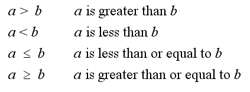The following are a set of properties for inequalities: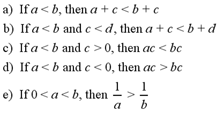The solution set of an inequality is the set of numbers for which the inequality is true. Two inequalities are equivalent if they have the same solution sets.

# Examples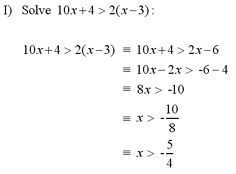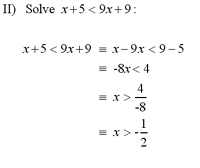Note: For examples of graphing an inequality, see questions #2 and #3 in Additional Examples at the bottom of the page.

# Absolute Value

The absolute value of a real number x can be thought of as the distance from 0 to x on the real number line. Absolute value, denoted |x|, is defined as follows: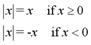Note: Absolute value, |x| is always nonnegative, since it is a distance on the real number line.

# Properties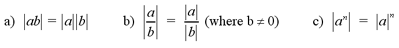# Examples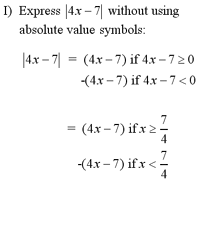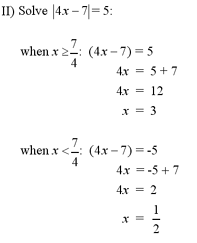# Absolute Value & Inequalities

The properties listed below describe how to solve inequalities that contain absolute values.

# Properties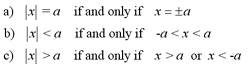# Examples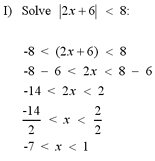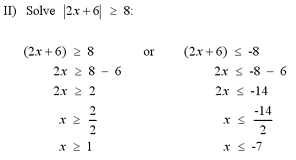Note: For a more complex example of solving an absolute inequality, see question #1 in the Additional Examples section below.

1 | Solve the inequality
2 | Graph the region determined by the inequality
3 | Graph the region determined by the parabolic inequality

| Top of Page |

COURSE HOMEPAGES
MATH 1036
MATH 1037

FACULTY HOMEPAGES
Alex Karassev
Ted Chase